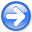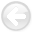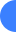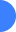Migration velocity analysis based on linearization of the two-way wave equationNext: Introduction Up: Reproducible Documents

### Abstract:

Wave equation migration velocity analysis (WEMVA) is a family of techniques that aims to improve the subsurface velocity model by minimizing the residual in image space. This process is performed iteratively by linearizing the imaging operator in order to relate image perturbations to model updates. This linearization is conventionally based on the one-way wave equation, which has some pitfalls in terms of accuracy and ability to image certain wavepaths in complex geology. We present a formulation of WEMVA based on the two-way wave equation which can improve the accuracy of the model estimate. There are two approximations used to linearize this operator. First is the Born approximation and the second envolves dropping the second order slowness perturbation term. In this paper, we show preliminary results of using the two-way based WEMVA, as well as the resolution matrix of the operator.

2010-11-26